#Function Repository Resource:

# MeanCI

Estimate the confidence interval for the population mean

Contributed by: Wolfram Research
 ResourceFunction["MeanCI"][list] gives a confidence interval for the population mean estimated from list.

## Details and Options

ResourceFunction["MeanCI"][list] gives a confidence interval {min,max} centered at Mean[list].
If the variance is assumed to be known, ResourceFunction["MeanCI"] is based on the normal distribution.
If the variance is not assumed to be known, ResourceFunction["MeanCI"] is based on the Student t distribution with Length[list]-1 degrees of freedom.
The following options can be given:
 0.95 probability associated with a confidence interval KnownVariance variance of population

## Examples

### Basic Examples

A 95% confidence interval for the population mean:

 In:=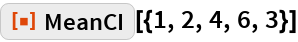Out=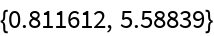### Options

#### ConfidenceLevel

A 99% confidence interval:

 In:=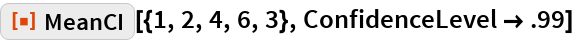Out=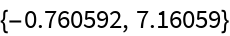#### KnownVariance

A 95% confidence interval assuming the population variance is 1:

 In:=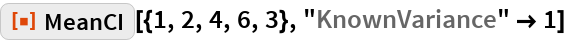Out=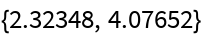## Requirements

Wolfram Language 11.3 (March 2018) or above